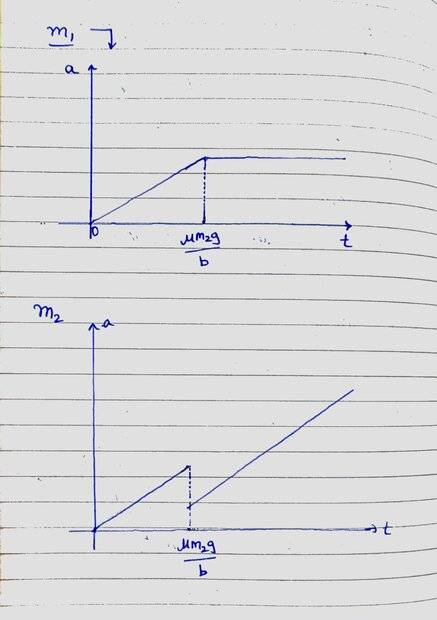# Just a quick graph question -- Time varying force applied to two blocks on a surface

Differentiate it
Homework Statement:
Acceleration of Blocks Due to a Time Varying Force: A block m, is placed on smooth ground over which another mass m, is placed. Friction coefficient between m, and m₂ is u. Now a time varying horizontal external force F = bt is applied on m2. Find acceleration of block with time and plot acceleration v/s time graph of both blocks.
Relevant Equations:
F = maI have attempted this question - Are the graphs correct?

#### Attachments

Last edited:

Gold Member
Is ##m_2## sitting on top of ##m_1##? It isn't clear in the problem statement.

•berkeman
Homework Helper
2022 Award
What causes the discontinuity?

Gold Member
1) What is the reasoning that you expect a drop in acceleration for ##m_2## once the force ##F## exceeds the static friction between the masses (think about the change in force acting on each of the masses from static to kinetic friction)?

2) The slope of the acceleration vs time before and after the discontinuity appear parallel to me, is that drawn to scale (they won't be parallel)?

3) For the same reason in (1), how does that effect the graph for ##m_1## (there is a missing discontinuity there)?

Last edited:
•hutchphd
Differentiate it
1) What is the reasoning that you expect a drop in acceleration for ##m_2## once the force ##F## exceeds the static friction between the masses?

2) The slope of the acceleration vs time before and after the discontinuity appear parallel to me, is that drawn to scale?
Yes, it is supposed to be parallel Here is my working if you want to take a look

#### Attachments

Gold Member
So, you think the slope of ##a_2(t)## before and after ##t = \frac{\mu m_2g}{b}## are equal. Hmmm.

Before the force of static friction is exceeded what is the acceleration ##a_2(t)## of ##m_2##? What is the acceleration ##a_1(t)## of ##m_1##?

Differentiate it
So, you think the slope of ##a_2(t)## before and after ##t = \frac{\mu m_2g}{b}## are equal. Hmmm.

Before the force of static friction is exceeded what is the acceleration ##a_2(t)## of ##m_2##? What is the acceleration ##a_1(t)## of ##m_1##?
Both are the same: bt/m1

Gold Member
Both are the same:
The first part is correct. They are both the same.
bt/m1
The second part is not.

•hutchphd
Differentiate it
The first part is correct. They are both the same.

The second part is not.
•